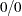Calling Functions in the R Language
 Special Numeric Values

The R language has four symbols that are used to represent special numerical values.

• The symbol NA represents a missing value.

• The symbol Inf represents positive infinity.

• The symbol –Inf represents positive infinity.

• The symbol NaN represents a "NaN," which is a floating-point value that represents an undefined value such as the result of the division.

The SAS language has 28 symbols that are used to represent special numerical values.

• The symbol . represents a generic missing value.

• The symbols .A.Z and ._ are also missing values. Some applications use .I to represent positive infinity and use .M to represent negative infinity.

The following table shows how special numeric values in R are converted to SAS missing values:

Value in R

SAS Missing Value

Inf

.I

–Inf

.M

NA

.

NaN

.

The following table shows how SAS missing values are converted when data are transferred to R:

SAS Missing Value

Value in R

.I

Inf

.M

–Inf

All others

NAPrevious Page | Next Page | Top of Page